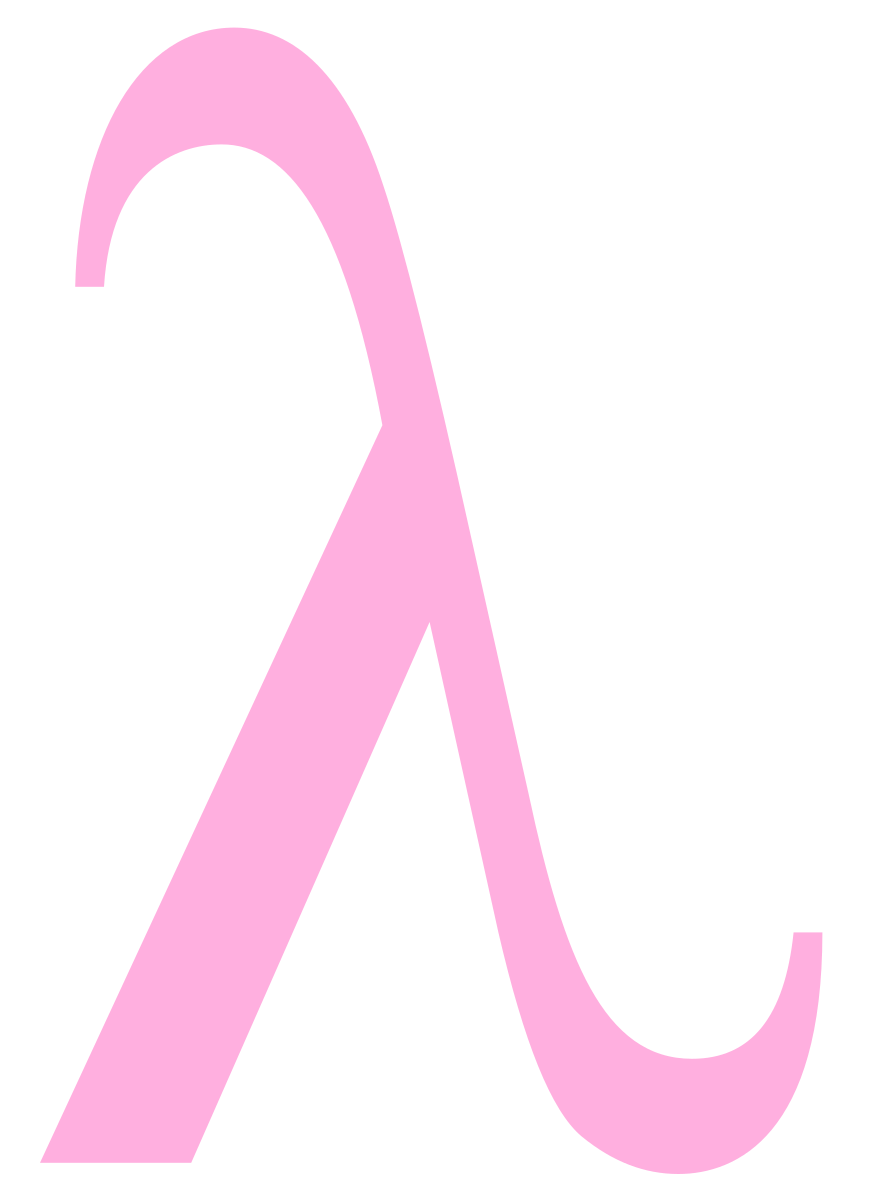# Lambdas For C — Sort Of

A lot of programming languages these days feature lambda functions, or what I would be just as happy to call anonymous functions. Some people make a big deal out of these but the core idea is very simple. Sometimes you need a little snippet of code that you only need in one place — most commonly, as a callback function to pass another function — so why bother giving it a name? Depending on the language, there can be more to it that, especially if you get into closures and currying.

For example, in Python, the map function takes a function as an argument. Suppose you have a list and you want to capitalize each word in the list. A Python string has a capitalize method and you could write a loop to apply it to each element in the list. However, `map` and a lambda can do it more concisely:

`map(lambda x: x.capitalize(), ['madam','im','adam'])`

The anonymous function here takes an argument x and calls the capitalize method on it. The map call ensures that the anonymous function is called once for each item.

Modern C++ has lambda expressions. However, in C you have to define a function by name and pass a pointer — not a huge problem, but it can get messy if you have a lot of callback functions that you use only one time. It’s just hard to think up that many disposable function names. However, if you use gcc, there are some nonstandard C features you can use to get most of what you want out of lambda expressions.

## Why GCC?You have to use gcc, because this trick uses two nonstandard language features. First, gcc allows nested functions. In addition, you can use statement expressions. That is an expression that can do everything a function can.

These two things, combined with some abuse of the preprocessor, will let you stuff your function code right where you need it and relieve you of having to name each and every little function you write just to pass to another subroutine.

If you use another compiler, it might not — in fact, probably doesn’t — have these features. In the end, though, you could always just use a proper function with a pointer. This is a bit nicer.

## Let’s StartI created a simple example of a case where you might want to use a lambda in C. You can find it on GitHub. There’s an array of floating point numbers, `thelist`, and two functions that process the list. One averages the list after multiplying each value by 2. The other does the same task but divides each entry by 3 first.

A contrived example, to be sure, but easy enough to understand. If you study the code, the two averaging functions, `average2x` and `averagethird`, are almost the same. The only difference is the one point where the math is different.

Now look at this version. Here, the `average_apply` function does all the averaging work, but it requires a function pointer, `fn`, to do the math operation you want. There are two functions to work out the math:

```float twox(float x) { return 2*x; }
float xdiv3(float x) { return x/3; }```

Then making the call is easy:

```printf("%fn", average_apply(twox));
printf("%fn", average_apply(xdiv3));```

## Of Course…

Those little snippets of code now need names and we won’t really use them anywhere else. That’s the perfect spot for a lambda. You can put this in a header file (although I just left it at the top of the file for this example):

```#define lambda(lambda\$_ret, lambda\$_args, lambda\$_body)
({
lambda\$_ret lambda\$__anon\$ lambda\$_args
lambda\$_body
&amp;lambda\$__anon\$;
})```

This takes advantage of the gcc features I mentioned and the fact that gcc will let you use dollar signs in identifiers. That means that you might need to pass `-std=gnu99` on the gcc command line to make this work properly. This should do it:

`gcc -o clambda2 --std=gnu99 clambda2.c`

Now the calls just put the functions right in line:

```printf("%fn", average_apply(lambda(float,(float x),{ return 2*x; })));
printf("%fn", average_apply(lambda(float,(float x),{ return x/3.0; })));```

By the way, I realize you could just pass a factor of 2 or 0.3333 to one function, but that’s not the point. The functions could be as complex as you like. Like most programming problems, there are many ways to solve this.

## Caveats

There are caveats. You can’t depend on the lambda having a scope beyond the enclosing function so an asynchronous callback would not work. Accessing local variables in the enclosing scope is iffy. Consider this code:

```int factor=10;
printf("%fn",average_apply(lambda(float,(float x),{ return x/factor; })));```

This compiles and ought to work. It does work for this online compiler. However, using the Linux system for Windows 10, the same code would seg fault. In fact, if you didn’t set the gcc -O2 option, the other examples would seg fault, too. On my Linux boxes, it works fine. I can’t tell if this is a bug in Windows or if Linux is just covering up something wrong. However, it seems like if it compiles it ought to work and — mostly — it does.

## In Practice

Granted, you don’t have to have lambdas with C. Even if you want them, they aren’t very portable. But it is still interesting to see how some gcc-specific compiler features work and it is also an interesting intellectual exercise to try to match it.

The technique is not new. If you search, you can find quite a few postings in various places with the same idea, although each one has small variations. Of course, in C++ of recent vintage you can use lambdas with no problem. By the way, lambdas are all the rage for cloud computing, too, and it is a similar idea. A little function that just runs without having to wait on a server for a request.

GCC logo GFDL.